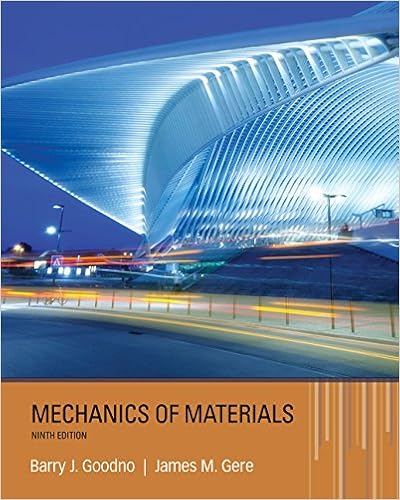# Which of the following statements is true a

• Notes
• 55
• 100% (1) 1 out of 1 people found this document helpful

This preview shows page 16 - 20 out of 55 pages.

##### We have textbook solutions for you!
The document you are viewing contains questions related to this textbook.The document you are viewing contains questions related to this textbook.
Chapter 4 / Exercise 4.3-9
Mechanics of Materials
Gere/GoodnoExpert Verified
7) Which of the following statements is true? a. Biomaterials are materials that mainly contain hydrocarbons b. Biomaterials have their origins in agricultural products only c. Biomaterials should not produce toxic substances in human body d. Biomaterials easily degrade when exposed to sun light
8) Properties of Iron can be changed over a very wide range by imparting proper heat treatment
9) Properties of materials depend upon their structure and hence do not dependent on the processes undergone during their production
10) If a material is squeezed between a vise;
##### We have textbook solutions for you!
The document you are viewing contains questions related to this textbook.The document you are viewing contains questions related to this textbook.
Chapter 4 / Exercise 4.3-9
Mechanics of Materials
Gere/GoodnoExpert Verified
11) If a material is subjected to torsion; a. it experiences sheer stresses b. it experiences tensile stresses c. it experiences compressive stresses
12) If a long bar having a rectangular cross section (a x b) and length l experiences a force F along its length, the normal stress it endures is defined as:
13) If a long bar having a rectangular cross section (a x b) and length l experiences torsion forces along its length, the shear stress “tau” acts on the area:
14) Engineering stress on a body deformed by the application of a force is defined as:
15) True stress on a body deformed by the application of a force is defined as: a. Force divided by the actual cross sectional area b. Force multiplied by the actual cross sectional area c. Force multiplied by the original cross sectional area d. Force divided by the original cross sectional area
16) Stresses generated due to the application of hydrostatic pressure (such as body submerged in ocean) are always tensile in nature:
17) Units of stress are:
18) Units of strain are:
19) The relationship between stress and strain is:
a. Stress is directly proportional to strain b. Stress is inversely proportional to strain c. Stress is proportional to the square of strain
•••